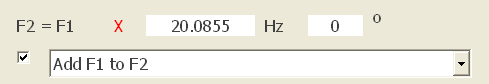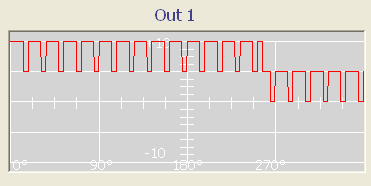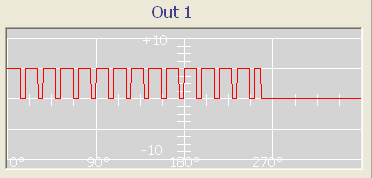Simulating F100 vbackfreq command with Spooky for scalar octaves as background frequencies.

Jeff Sutherland (Frequency Foundation) is extensively using scalar octaves in his frequency sets as background frequencies as he claims they increase the efficiency and cut the time needed to eliminate pathogens. You can read more about it here:

https://web.archive.org/web/20060220182647/http://www.frequencyresearch.org:80/2005/05/where-do-scalar-octave-frequencies.html

About a year ago I abandoned binary octaves of frequencies, i.e. dividing by 2 to get lower octaves of primary frequencies that are usable on plasma devices that only generate frequencies up to 10000 hertz. I found a method of calculating scalar octaves that I now use consistently. Simply divide or multiply by exp(3), exp(6), or exp(9). In the Excel spreadsheet language you can calculate the scalar octave of A1 by inserting =A1/exp(3) in another cell, and so forth.

Binary octaves do not work as well as primary frequencies. In general, scalar octaves do work as well as primary frequencies. In fact, they cut the time required to kill a pathogen by more than 50% over binary octaves. This has been confirmed in more than a thousand experiments.
I tried to incorporate these scalar octaves with the frequency sets I run with Spooky and found a way to do that:

Jeff Sutherland uses the F100 progamming language for his frequency sets. To add scalar octaves as background frequency he uses the vbackfreq command in virtually all his frequency sets:

vbackfreq b 0.049787068 0 71.5

This sets a variable background frequency based on the main frequency. In that case the background frequency is equal to the main frequency multiplied by 0.049787068 and has a duty cycle of 71.5%. So basically two frequencies are mixed. The main (kill) frequency and its scalar octave.

Why exactly 0.049787068?

Because:

e^3 = 20.085536923
1 / (e^3) = 0.049787068

So for example if the main kill frequency is 100,000 Hz, this would add a background frequency with 4978.7068 Hz. The main kill frequency is essentially pulsed by another related frequency which is supposed to increase efficiency compared to just running the main kill frequency without pulse / background frequency.

In Spooky there is no way to add a variable background frequency with the factor 0.049787068 to the main frequency by using the in-built F1/F2 function because only multipliers greater than 1.0 are supported. However it is also possible to do the reverse: use the background frequency (original frequency x 0.049787068) as the main frequency and multiply it with e^3 = 20.085536923 to arrive back at the original frequency.

So for the above example we could use

F1 = 4978.7068 Hz
F2 = F1 x 20.085536923 = 100,000 Hz

For a square wave with 71% Duty Cycle and offset 100% the resulting output would look like this:However this is not exactly the output that would be produced by a F165 generater and the vbackfreq command that Jeff Sutherland uses. F1 and F2 need to be mixed with a logical AND function. This is not possible using the Spooky software. For this purpose I generated a custom waveform that represents square wave with 100% offset and 71.5% duty cycle mixed with its own 3rd scalar octave using logical AND:SquareScalar.csv

Using this waveform together with the original kill frequencies multiplied by the factor 0.049787068 should lead to the same output a F165 generator would give when using the above mentioned vbackfreq.

100,000 Hz
220,000 Hz
530,000 Hz

You would now have to use the following frequencies:

4978.7068 Hz
10953.15496 Hz
26387.14604 Hz

In addition with the new waveform (which acts as a multiplier of 20.085536923) this will result in the original frequencies along with the 3rd scalar octave as background frequency.

So far I was not able to verify the efficiency of using scalar octaves as I do not have an objective way to measure the impact of frequencies, but maybe some other members find it useful to experiment with it.